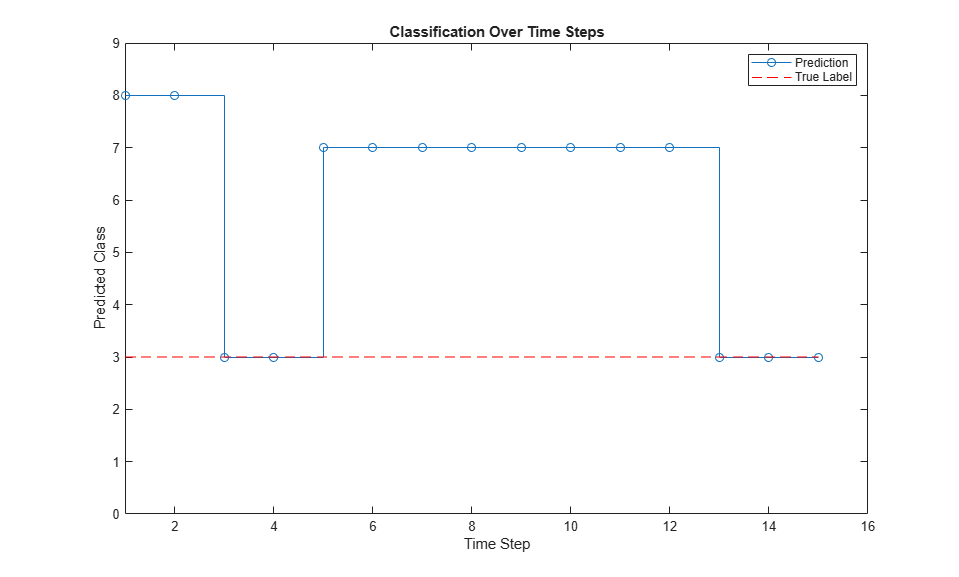Classify and Update Network State in Simulink

This example shows how to classify data for a trained recurrent neural network in Simulink® by using the Stateful Classify block. This example uses a pretrained long short-term memory (LSTM) network.

Load JapaneseVowelsNet, a pretrained long short-term memory (LSTM) network trained on the Japanese Vowels data set as described in  and . This network was trained on the sequences sorted by sequence length with a mini-batch size of 27.

View the network architecture.

net.Layers
ans =

5x1 Layer array with layers:

1   'sequenceinput'   Sequence Input          Sequence input with 12 dimensions
2   'lstm'            LSTM                    LSTM with 100 hidden units
3   'fc'              Fully Connected         9 fully connected layer
4   'softmax'         Softmax                 softmax
5   'classoutput'     Classification Output   crossentropyex with '1' and 8 other classes

Load the Japanese Vowels test data. XTest is a cell array containing 370 sequences of dimension 12 of varying length. YTest is a categorical vector of labels "1","2",..."9", which correspond to the nine speakers.

[XTest,YTest] = japaneseVowelsTestData;
X = XTest{94};
numTimeSteps = size(X,2);

The Simulink model for classifying data contains a Stateful Classify block to predict the labels and MATLAB Function blocks to load the input data sequence over the time steps.

open_system('StatefulClassifyExample');Configure Model for Simulation

Set the model configuration parameters for the input blocks and the Stateful Classify block.

set_param('StatefulClassifyExample/Input','Value','X');
set_param('StatefulClassifyExample/Index','uplimit','numTimeSteps-1');
set_param('StatefulClassifyExample/Stateful Classify','NetworkFilePath','JapaneseVowelsNet.mat');
set_param('StatefulClassifyExample','SimulationMode','Normal');

Run the Simulation

To compute responses for the JapaneseVowelsNet network, run the simulation. The prediction labels are saved in the MATLAB® workspace.

out = sim('StatefulClassifyExample');

Plot the predicted labels in a stair plot. The plot shows how the predictions change between time steps.

labels = squeeze(out.YPred.Data(1:numTimeSteps,1));

figure
stairs(labels, '-o')
xlim([1 numTimeSteps])
xlabel("Time Step")
ylabel("Predicted Class")
title("Classification Over Time Steps")Compare the predictions with the true label. Plot a horizontal line showing the true label of the observation.

trueLabel = double(YTest(94));
hold on
line([1 numTimeSteps],[trueLabel trueLabel], ...
'Color','red', ...
'LineStyle','--')
legend(["Prediction" "True Label"])
axis([1 numTimeSteps+1 0 9]);M. Kudo, J. Toyama, and M. Shimbo. "Multidimensional Curve Classification Using Passing-Through Regions." Pattern Recognition Letters. Vol. 20, No. 11–13, pages 1103–1111.

 UCI Machine Learning Repository: Japanese Vowels Dataset. https://archive.ics.uci.edu/ml/datasets/Japanese+Vowels